JEE  >  BITSAT Logical Reasoning Test - 4

# BITSAT Logical Reasoning Test - 4

Test Description

## 10 Questions MCQ Test BITSAT Mock Tests Series & Past Year Papers | BITSAT Logical Reasoning Test - 4

BITSAT Logical Reasoning Test - 4 for JEE 2022 is part of BITSAT Mock Tests Series & Past Year Papers preparation. The BITSAT Logical Reasoning Test - 4 questions and answers have been prepared according to the JEE exam syllabus.The BITSAT Logical Reasoning Test - 4 MCQs are made for JEE 2022 Exam. Find important definitions, questions, notes, meanings, examples, exercises, MCQs and online tests for BITSAT Logical Reasoning Test - 4 below.
Solutions of BITSAT Logical Reasoning Test - 4 questions in English are available as part of our BITSAT Mock Tests Series & Past Year Papers for JEE & BITSAT Logical Reasoning Test - 4 solutions in Hindi for BITSAT Mock Tests Series & Past Year Papers course. Download more important topics, notes, lectures and mock test series for JEE Exam by signing up for free. Attempt BITSAT Logical Reasoning Test - 4 | 10 questions in 10 minutes | Mock test for JEE preparation | Free important questions MCQ to study BITSAT Mock Tests Series & Past Year Papers for JEE Exam | Download free PDF with solutions
 1 Crore+ students have signed up on EduRev. Have you?
BITSAT Logical Reasoning Test - 4 - Question 1

### Choose the word which is least like the other words in the group .

BITSAT Logical Reasoning Test - 4 - Question 2

### Complete the analogous pair. Premium : Profit :: Discount : ?

BITSAT Logical Reasoning Test - 4 - Question 3

### Group the following figures into three classes on the basis of identical properties.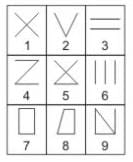BITSAT Logical Reasoning Test - 4 - Question 4

Find the missing character.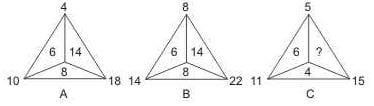Detailed Solution for BITSAT Logical Reasoning Test - 4 - Question 4

In fig. (A), 10 - 4 = 6, 18 - 10 = 8, 18 - 4 = 14.
In fig. (B), 14 - 8 = 6, 22 - 14 = 8, 22 - 8 = 14.
In fig. (C), 11 - 5 = 6, 15 - 11 = 4.
So, missing number = 15 - 5 = 10.

BITSAT Logical Reasoning Test - 4 - Question 5

In this letter series, some of the letters are missing. Choose the correct letter given below -
aa _ ab _ _ aaa _ a

Detailed Solution for BITSAT Logical Reasoning Test - 4 - Question 5 It is following some definite pattern ie. (aaaa)(b)(aaaaa)(b)(a..... Here it is clear that the number of an's is increasing with b acting as separation, Hence A is the correct option.
BITSAT Logical Reasoning Test - 4 - Question 6

In this letter series, some of the letters are missing. Choose the correct letter given below -
a _ bccb _ ca _ cca _ baab _ c

BITSAT Logical Reasoning Test - 4 - Question 7

Select the correct mirror-image of the Figure (X) from amongst the given alternatives.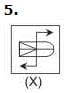BITSAT Logical Reasoning Test - 4 - Question 8

Select the correct mirror-image of the Figure (X) from amongst the given alternatives.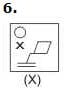BITSAT Logical Reasoning Test - 4 - Question 9

Each of the following questions consists of five Problem figures marked 1,2,3,4 and 5 followed by five Answer figures marked A,B,C,D and E . Select a figure from the Answer figures which will continue the same series as given in the Problem figures.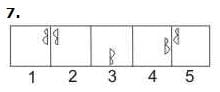Detailed Solution for BITSAT Logical Reasoning Test - 4 - Question 9

Explanation : The symbol gets vertically inverted and laterally inverted alternately. It also moves in ACW direction through distances equal to two half-sides (of square boundary) and three half-sides alternately.

BITSAT Logical Reasoning Test - 4 - Question 10

Each of the following questions consists of five Problem figures marked 1,2,3,4 and 5 followed by five Answer figures marked A,B,C,D and E . Select a figure from the Answer figures which will continue the same series as given in the Problem figures.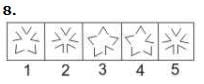## BITSAT Mock Tests Series & Past Year Papers

3 videos|15 docs|70 tests
 Use Code STAYHOME200 and get INR 200 additional OFF Use Coupon Code
Information about BITSAT Logical Reasoning Test - 4 Page
In this test you can find the Exam questions for BITSAT Logical Reasoning Test - 4 solved & explained in the simplest way possible. Besides giving Questions and answers for BITSAT Logical Reasoning Test - 4, EduRev gives you an ample number of Online tests for practice

## BITSAT Mock Tests Series & Past Year Papers

3 videos|15 docs|70 tests